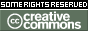REPOZYTORIUM UNIWERSYTETU
W BIAŁYMSTOKUProszę używać tego identyfikatora do cytowań lub wstaw link do tej pozycji: `http://hdl.handle.net/11320/6829`
 Tytuł: Introduction to Stochastic Finance: Random Variables and Arbitrage Theory Autorzy: Jaeger, Peter Słowa kluczowe: random variablearbitrage theoryrisk-neutral measure Data wydania: 2018 Data dodania: 20-sie-2018 Wydawca: DeGruyter Open Źródło: Formalized Mathematics, Volume 26, Issue 1, Pages 1–9 Abstrakt: Using the Mizar system , , we start to show, that the Call-Option, the Put-Option and the Straddle (more generally defined as in the literature) are random variables (, p. 15), see (Def. 1) and (Def. 2). Next we construct and prove the simple random variables (, p. 14) in (Def. 8). In the third section, we introduce the definition of arbitrage opportunity, see (Def. 12). Next we show, that this definition can be characterized in a different way (Lemma 1.3. in , p. 5), see (17). In our formalization for Lemma 1.3 we make the assumption that ϕ is a sequence of real numbers (there are only finitely many valued of interest, the values of ϕ in Rd). For the definition of almost sure with probability 1 see p. 6 in . Last we introduce the risk-neutral probability (Definition 1.4, p. 6 in ), here see (Def. 16). We give an example in real world: Suppose you have some assets like bonds (riskless assets). Then we can fix our price for these bonds with x for today and x · (1 + r) for tomorrow, r is the interest rate. So we simply assume, that in every possible market evolution of tomorrow we have a determinated value. Then every probability measure of Ωfut1 is a risk-neutral measure, see (21). This example shows the existence of some risk-neutral measure. If you find more than one of them, you can determine – with an additional conidition to the probability measures – whether a market model is arbitrage free or not (see Theorem 1.6. in , p. 6.) A short graph for (21): Suppose we have a portfolio with many (in this example infinitely many) assets. For asset d we have the price π(d) for today, and the price π(d) (1 + r) for tomorrow with some interest rate r > 0. Let G be a sequence of random variables on Ωfut1, Borel sets. So you have many functions fk : {1, 2, 3, 4}→ R with G(k) = fk and fk is a random variable of Ωfut1, Borel sets. For every fk we have fk(w) = π(k)·(1+r) for w {1, 2, 3, 4}. Today Tomorrow only one scenario {w21={1,2} w22={3,4} for all d∈N holds π(d) {fd(w)=G(d)(w)=π(d)⋅(1+r), w∈w21 or w∈w22, r>0 is the interest rate. Here, every probability measure of Ωfut1 is a risk-neutral measure. Afiliacja: Siegmund-Schacky-Str. 18a, 80993 Munich, Germany URI: http://hdl.handle.net/11320/6829 DOI: 10.2478/forma-2018-0001 ISSN: 1426-2630 e-ISSN: 1898-9934 Typ Dokumentu: Article Występuje w kolekcji(ach): Formalized Mathematics, 2018, Volume 26, Issue 1

Pliki w tej pozycji:
Plik Opis RozmiarFormat
Pozycja ta dostępna jest na podstawie licencji Licencja Creative Commons CCL